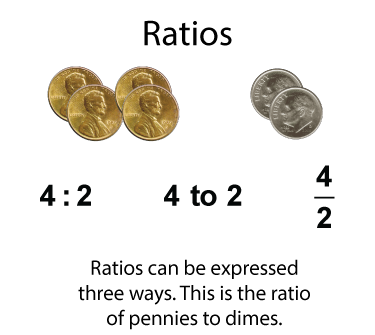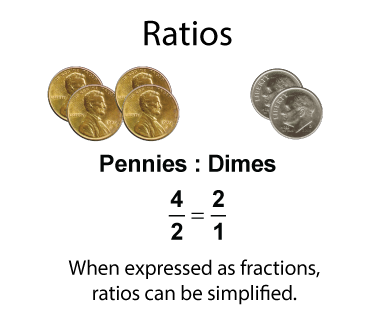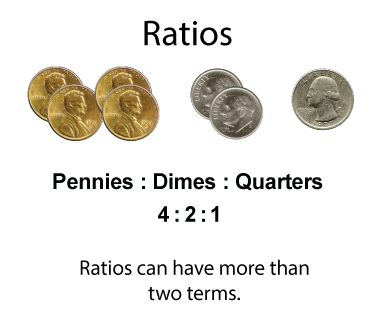# Closed Captioned Video: Ratios: Fractional Ratios

## What Are Ratios?

A ratio is the relationship between two or more quantities among a group of items. Let's look at an example.Here is a collection of coins. The ratio of pennies to dimes can be expressed as a ratio, and a ratio can be written in three different, but equivalent ways.

When expressed as fractions, ratios can then be rewritten as fractions in simplest form.For some groupings, you can write a ratio that includes three terms.Ratios usually include similar types of objects. Here is a collection of different types of balls.Rates are a special type of ratio. Rates involve different types of quantities. Here is an example of a rate involving cost and weight.Video Tutorial: Ratios: Fractional Ratios. In this video look at ratios of the form a:b and a:b:c, where the terms in the ratio are fractions. Techniques are shown for converting fractional ratios to whole number ratios.

## Video Transcripts

### This video has a transcript available. To see the complete collection of video transcripts, click on this link.

Common Core Standards CCSS.MATH.CONTENT.6.RP.A.2, CCSS.MATH.CONTENT.6.RP.A.1, CCSS.MATH.CONTENT.7.RP.A.1 4.5 minutes 6 - 8 Algebra     • Ratios, Proportions, and Percents         • Ratios and Rates 2020 ratios, ratios with fractions, video tutorial, Closed Captioned Video